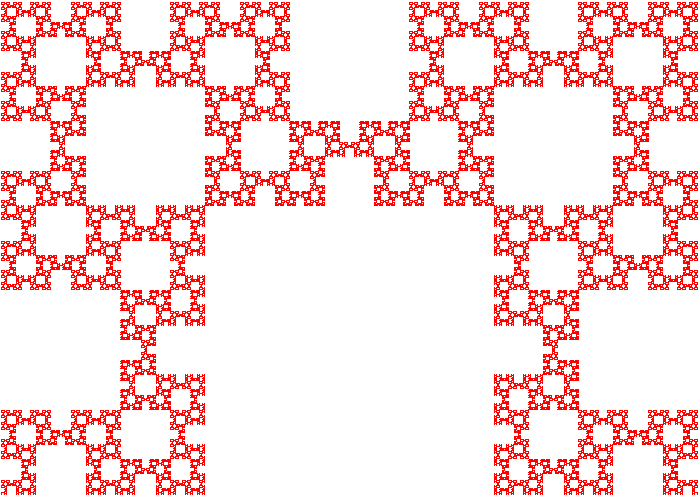09 July 2009

Check out this article for a lot of interesting properties of this fractal and its relation to the Fibonacci sequence. F# code follows after the image.Fibonacci word fractal

``````open System
open System.Drawing
open System.Drawing.Imaging

let right = Math.PI / 2.0
let left  = 3.0 * Math.PI / 2.0

type Alphabet =
|K of float
|Q of float
|R of float
|L of float
|T of float

let fibonacci_word = function
|L(x) -> [T(left) ; R(x); T(right); L(x); K(x); L(x); T(right); R(x); T(left) ]
|R(x) -> [T(right); L(x); T(left) ; R(x); Q(x); R(x); T(left) ; L(x); T(right)]
|K(x) -> [L(x)]
|Q(x) -> [R(x)]
|T(x) -> [T(x)]

let rec internal applyN f n x =
if n = 0 then x else f (applyN f (n-1) x)

type ITurtle =
abstract draw_forward : float -> unit
abstract move_forward : float -> unit
abstract turn         : float -> unit

let interpreter (turtle: ITurtle) = function
|L(x) -> turtle.draw_forward(x)
|R(x) -> turtle.draw_forward(x)
|K(x) -> turtle.move_forward(x)
|Q(x) -> turtle.move_forward(x)
|T(x) -> turtle.turn(x)

let rotate (x,y) theta =
let x' = x * cos theta - y * sin theta
let y' = x * sin theta + y * cos theta
(x',y')

let Turtle(screen:Graphics) =
let x = ref 1.0
let y = ref 500.0
let dx = ref 1.0
let dy = ref 0.0
{ new ITurtle with
member t.draw_forward(scale) = screen.DrawLine( new Pen(Color.Red),
PointF(float32 !x,float32  !y),
PointF(float32(!x + (!dx*scale)),
float32( !y + (!dy*scale))))
t.move_forward(scale)
member t.move_forward(scale) = x := !x + (!dx*scale)
y := !y + (!dy*scale)
member t.turn(theta)    = let dx',dy' = rotate (!dx,!dy) (theta)
dx := dx'
dy := dy'

};;

let bitmap = new Bitmap(1000,1000)

let turtle = Turtle(Graphics.FromImage(bitmap))

List.iter (interpreter turtle) (applyN (List.collect fibonacci_word) 8 [L(0.5)])

bitmap.Save("fibonacci_word.bmp")

``````